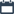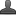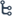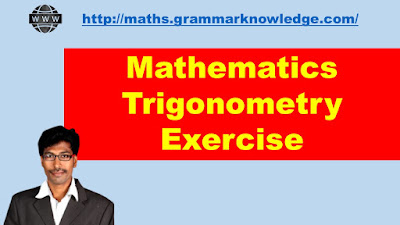## Friday, October 2, 2020

•October 02, 2020
••,
•1 comment

## Mathematics trigonometry Exercise - 11.1

Hi friends and my dear students! In this post, I have covered Andhra Pradesh SSC(10th class) Mathematics trigonometry Exercise - 11.1 Solutions part-1. After Reading Mathematics trigonometry Exercise, Please do share it with your friends. You canMathematics Trigonometry Exercise

6. If ∠a and ∠x are acute angles   such that Cos A = Cos X then such that   ∠a = ∠x

Sol: Given that   Cos A = Cos X   ------   (1)

We have   Cos A =AB/AC and   Cos X = XY /XY

AB/AC = XY/XZ   From  Eg (1)

AB/AC = XY/XZ = k ------   (2)

From ⧍ABC

BC 2 =AC 2-AB 2

BC 2 = AC 2   - (k AC) 2

BC 2=AC 2   -   k 2 AC 2

BC 2=AC 2   (1- k 2)

BC =AC 2     (1- k 2)

BC =AC 2   (1- k 2)

BC = AC (1- k 2)

From ⧍ XYZ

YZ 2 =AC 2-XY 2

YZ 2 = XZ 2   - (k XZ) 2

YZ 2= XZ 2   -   k 2 XZ 2

YZ 2= XZ 2   (1- k 2)

YZ = XZ 2   (1- k 2)

YZ = XZ    (1- k 2)

BC/ YZ = AC (1- k 2)/ XZ    (1- k 2)

BC/ YZ = AC/ XZ   ------   (3)

From (2) & (3) Equations

Hence   AB/XY= BC/ YZ = AC/ XZ

⧍ ABC ~ ⧍ XYZ (   .  ̇. ∠A =∠X   )

7. Cot 𝜽 =7/8, evaluate (1)   (1+sin 𝜽) (1-sin 𝜽) / (1+cos 𝜽) (1-cos 𝜽)   (2) (1+sin 𝜽)/ Cos 𝜽

Sol:   Cot 𝜽 =7/8

From ⧍ABC

AB 2= BC 2+AC 2

AB 272+82

AB 2= 49+64

AB 2=113

AB =113

2)(1+sin 𝜽) (1-sin 𝜽) /(1+cos 𝜽) (1-cos 𝜽)

= 1-sin 2 𝜽/1-cos 2 𝜽

= cos 2 𝜽 /sin 2 𝜽

= Cot 2 𝜽

= (BC/AB) 2

= (7/113) 2

= 72/(√113) 2

=49/113

(2)(1+sin 𝜽)/ Cos 𝜽

=1/Cos 𝜽+Sin 𝜽/Cos 𝜽

=Sec 𝜽+Tan 𝜽

=AB/BC+AC/BC

=113/ 7+8/7

=113+8/7

8. In a Right angle triangle ABC, Right angle is at B, Tan A =3 then find   the value of   1) Sin A Cos C + Cos A Sin C  2) Cos A Cos C - Sin A Sin C

Sol:    Given that   Tan A   = 3/1

(Hypotenuse) 2= (side) 2+ (side) 2

(Hypotenuse) 2(√3) 2 +1

=3+1

=4

1 1) Sin A Cos C + Cos A Sin C

=√3/2 ×√3/2+1/2×1/2

=3/4 +1/4

=4/4

=1

2) Cos A Cos C - Sin A Sin C

=1/2×√3/2 - 1/2×√3/2

=√3/4 -3/4

=0

#### 1 comment:

1.Trigonometry equations are always difficult to solve. Because you cannot learn and perform these types of equations in the first attempt. Doing it over and over again is the only way to learn trigonometry. Since childhood, trigonometry was the only subject that intimidated me when I appeared for the exam. But with the help of a lot of practice now it has become easier for me. I have learned trigonometry from online tutors. For more information contact h1 math tuition.